# DriftDiagnostics

Diagnostics information for batch drift detection

## Description

A `DriftDiagnostics` object stores the diagnostics information returned by the `detectdrift` function after it performs permutation testing for batch drift detection.

## Creation

Create a `DriftDiagnostics` object by using `detectdrift` to test for drift between baseline and target data sets.

## Properties

expand all

Baseline data set, specified as a numeric array, categorical array, or table.

Data Types: `double` | `categorical` | `table`

Indices of the categorical variables in the data, specified as a numeric array. If the data does not contain any categorical variables, then this property is empty (`[]`).

Data Types: `double`

95% confidence interval bounds for the estimated p-values of the variables, specified as a 2-by-k matrix of positive scalar values from 0 to 1, where k is the number of variables. The rows of `ConfidenceIntervals` correspond to the lower and upper bounds of the confidence intervals, respectively.

If you set `EstimatePValues` to `false` in the call to `detectdrift`, then the function does not compute the confidence interval bounds. In this case, `ConfidenceIntervals` property contains `NaN`s.

Data Types: `double`

Drift status for each variable, specified as a string array containing the possible values shown in this table.

Drift StatusCondition
DriftUpper < `DriftThreshold`
Warning`DriftThreshold` < Lower < `WarningThreshold` or `DriftThreshold` < Upper < `WarningThreshold`
StableLower > `WarningThreshold`

Lower and Upper are the lower and upper confidence interval bounds for an estimated p-value.

Data Types: `string`

Threshold to determine the drift status, specified as a scalar value from 0 to 1. If the upper bound of the confidence interval for the estimated p-value is below `DriftThreshold`, then the drift status is `Drift`.

Data Types: `double`

List of the metrics used by `detectdrift` to quantify the difference between the baseline and target data for each variable during permutation testing, specified as a string array.

Data Types: `string`

Metric values for the corresponding variables, specified as a row vector with the number of columns equal to the number of variables specified for drift detection. The metric corresponding to each variable is stored in the `Metrics` property.

Data Types: `double`

Multiple hypothesis testing correction, specified as either `"Bonferroni"` or `"FalseDiscoveryRate"`.

If you set `EstimatePValues` to `false` in the call to `detectdrift`, do not set the `MultipleTestCorrection` name-value argument because the function ignores it in this case.

Data Types: `string`

Drift status for the overall data estimated by `detectdrift` using the multiple test correction method in `MultipleTestCorrection`, specified as `"Drift"`, `"Warning"`, or `"Stable"`. Multiple test corrections provide a conservative estimate of the drift status when multiple variables are tested.

If you set `EstimatePValues` to `false` in the call to `detectdrift`, then the function does not populate `MultipleTestDriftStatus`.

Data Types: `string`

Number of permutation tests performed by `detectdrift` for each variable to determine the drift status for that variable, specified as an array of integer values.

If you set `EstimatePValues` to `false` in the call to `detectdrift`, then `NumPermutations` is a row vector of ones corresponding to the baseline and target data provided. The metric values are the initial computations that use the baseline and target data for each variable.

Data Types: `double`

Permutation testing results for each variable, specified as a k-by-1 table, where k is the number of variables. Each row corresponds to one variable and contains a 1-by-1 cell array of the metric values in a vector whose size is equal to the number of permutations for that variable. To access the metric values for the second variable, for example, use `DDiagnostics.PermutationResults{2,1}{1,1}`.

If you set `EstimatePValues` to `false` in the call to `detectdrift`, then `PermutationResults` contains only the initial metric values for each variable.

You can visualize the test results using `plotPermutationResults`.

Data Types: `table`

Estimated p-value for each variable, specified as a vector of scalar values from 0 to 1.

If you set `EstimatePValues` to `false` in the call to `detectdrift`, then `PValues` is a vector of `NaN`s.

Data Types: `double`

Target data set, specified as a numeric array, categorical array, or table.

Data Types: `single` | `double` | `categorical` | `table`

Variables specified for drift detection in the call to `detectdrift`, specified as a string array.

Data Types: `string`

Threshold to determine the warning status, specified as a scalar value from 0 to 1.

Data Types: `double`

## Object Functions

 `ecdf` Compute empirical cumulative distribution function (ecdf) for baseline and target data specified for data drift detection `histcounts` Compute histogram bin counts for specified variables in baseline and target data for drift detection `plotDriftStatus` Plot p-values and confidence intervals for variables tested for data drift `plotEmpiricalCDF` Plot empirical cumulative distribution function (ecdf) of a variable specified for data drift detection `plotHistogram` Plot histogram of a variable specified for data drift detection `plotPermutationResults` Plot histogram of permutation results for a variable specified for data drift detection `summary` Summary table for `DriftDiagnostics` object

## Examples

collapse all

`load humanactivity`

For details on the data set, enter `Description` at the command line.

Assign the first 250 observations as baseline data and the next 250 as target data for variables 1 to 15.

```baseline = feat(1:250,1:15); target = feat(251:500,1:15);```

Test for drift on all variables.

`DDiagnostics = detectdrift(baseline,target);`

Display a summary of the test results.

`summary(DDiagnostics)`
``` Multiple Test Correction Drift Status: Drift DriftStatus PValue ConfidenceInterval ___________ ______ ________________________ x1 "Drift" 0.001 2.5317e-05 0.0055589 x2 "Drift" 0.001 2.5317e-05 0.0055589 x3 "Drift" 0.001 2.5317e-05 0.0055589 x4 "Drift" 0.001 2.5317e-05 0.0055589 x5 "Drift" 0.001 2.5317e-05 0.0055589 x6 "Drift" 0.001 2.5317e-05 0.0055589 x7 "Drift" 0.001 2.5317e-05 0.0055589 x8 "Stable" 0.863 0.84012 0.88372 x9 "Stable" 0.726 0.69722 0.75344 x10 "Drift" 0.001 2.5317e-05 0.0055589 x11 "Stable" 0.496 0.46456 0.52746 x12 "Stable" 0.249 0.22247 0.27702 x13 "Drift" 0.001 2.5317e-05 0.0055589 x14 "Stable" 0.574 0.54267 0.60489 x15 "Warning" 0.094 0.076629 0.1138 ```

The summary table shows the drift status and estimated p-value for each variable tested for drift detection. You can also see the 95% confidence interval bounds for the p-values.

Plot drift status for variables `x10` to `x15`.

`plotDriftStatus(DDiagnostics,Variables=(10:15))`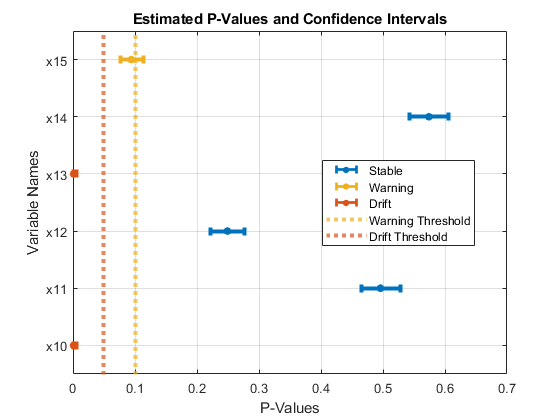Compute the ecdf values for variables `x13` and `x15`.

`E = ecdf(DDiagnostics,Variables=["x13","x15"])`
```E=2×3 table x F_Baseline F_Target ______________ ______________ ______________ x13 {501×1 double} {501×1 double} {501×1 double} x15 {501×1 double} {501×1 double} {501×1 double} ```

x contains the common domain over which `ecdf` computes the empirical cumulative distribution function for the baseline and target data of a variable. Access the common domain for `x13`.

`E.x{1}`
```ans = 501×1 0.0420 0.0420 0.0423 0.0424 0.0424 0.0425 0.0425 0.0426 0.0426 0.0426 ⋮ ```

Access the ecdf values for `x15` in the baseline data.

`E.F_Baseline{2}`
```ans = 501×1 0 0 0.0040 0.0080 0.0080 0.0080 0.0080 0.0080 0.0120 0.0120 ⋮ ```

Plot the ecdf values for variables `x13` and `x15`.

```tiledlayout(1,2) ax1 = nexttile; plotEmpiricalCDF(DDiagnostics,ax1,Variable="x13") ax2= nexttile; plotEmpiricalCDF(DDiagnostics,ax2,Variable="x15")```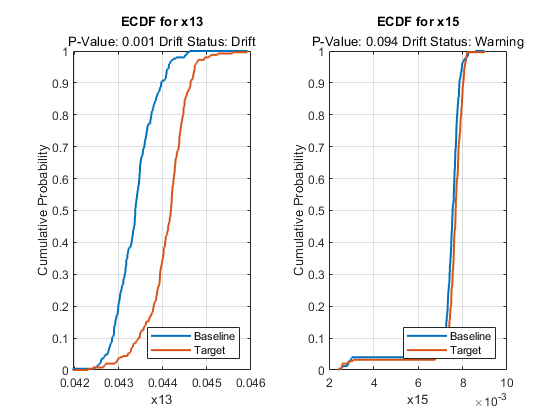You can also visualize the permutation test results for a variable. Plot the permutation results for variable `x13`.

```figure plotPermutationResults(DDiagnostics,Variable="x13")```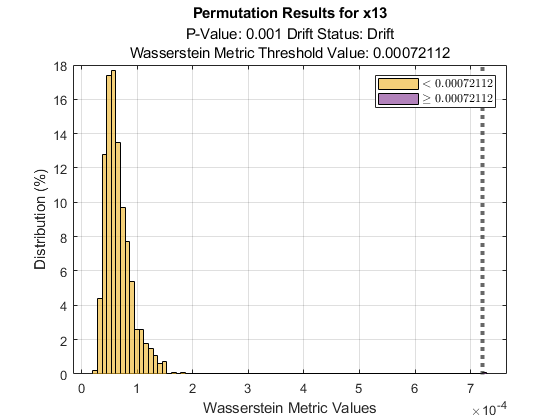The plot also shows the metric threshold value with a straight line. Based on the histogram of metric values obtained during permutation testing, the probability that a metric value being greater than the threshold value if the baseline and target data for variable x13 have the same distribution is very small. The plot also displays the estimated p-value, 0.001, and the drift status, `Drift`, below the plot title.

Generate baseline and target data with three variables, where the distribution parameters of the second and third variables change for the target data.

```rng('default') % For reproducibility baseline = [normrnd(0,1,100,1),wblrnd(1.1,1,100,1),betarnd(1,2,100,1)]; target = [normrnd(0,1,100,1),wblrnd(1.2,2,100,1),betarnd(1.7,2.8,100,1)];```

Compute the initial metrics for all variables between the baseline and target data without estimating the p-values.

`DDiagnostics = detectdrift(baseline,target,EstimatePValues=false)`
```DDiagnostics = DriftDiagnostics VariableNames: ["x1" "x2" "x3"] CategoricalVariables: [] Metrics: ["Wasserstein" "Wasserstein" "Wasserstein"] MetricValues: [0.2022 0.3468 0.0559] Properties, Methods ```

`detectdrift` computes only the initial metric value for each variable using the baseline and target data. The properties associated with permutation testing and p-value estimation are either empty or contain `NaN`s.

`summary(DDiagnostics)`
``` MetricValue Metric ___________ _____________ x1 0.20215 "Wasserstein" x2 0.34676 "Wasserstein" x3 0.055922 "Wasserstein" ```

`summary` function displays only the initial metric value and the metric used for each specified variable.

`plotDriftStatus` and `plotPermutationResults` do not produce plots and return warning messages when you compute metrics without estimating p-values. `plotEmpiricalCDF` and `plotHistogram` plot the ecdf and the histogram, respectively, for the first variable by default. They both return `NaN` for the p-value and drift status associated with the variable.

`plotEmpiricalCDF(DDiagnostics)`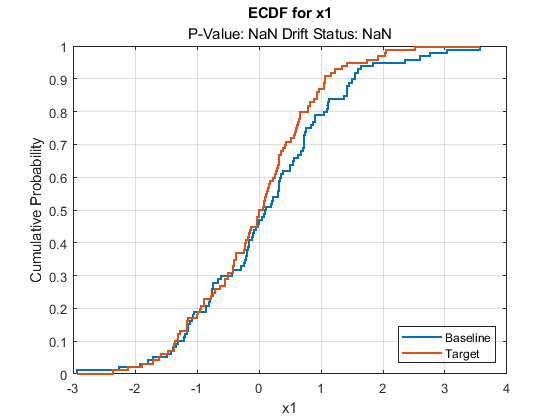`plotHistogram(DDiagnostics)`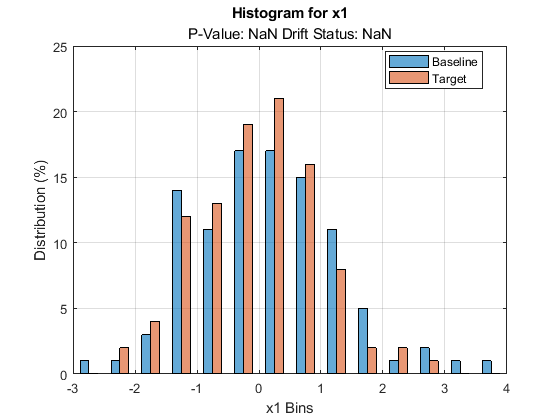## Version History

Introduced in R2022a